JMSLTM Numerical Library 7.2.0
com.imsl.math

## Class SVD

• ```public class SVD
extends Object```
Singular Value Decomposition (SVD) of a rectangular matrix of type `double`.

`SVD` is based on the LINPACK routine `SSVDC`; see Dongarra et al. (1979).

Let n be the number of rows in A and let p be the number of columns in A. For any
n x p matrix A, there exists an n x n orthogonal matrix U and a p x p orthogonal matrix V such that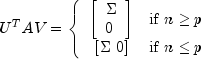where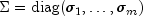, and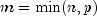. The scalars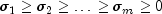are called the singular values of A. The columns of U are called the left singular vectors of A. The columns of V are called the right singular vectors of A.

The estimated rank of A is the number of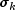that is larger than a tolerance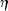. Ifis the parameter `tol` in the program, then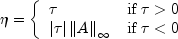The Moore-Penrose generalized inverse of the matrix is computed by partitioning the matrices U, V and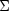as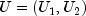,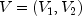and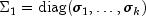where the "1" matrices are k by k. The Moore-Penrose generalized inverse is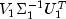.

Example
• ### Nested Class Summary

Nested Classes
Modifier and Type Class and Description
`static class ` `SVD.DidNotConvergeException`
The iteration did not converge
• ### Constructor Summary

Constructors
Constructor and Description
`SVD(double[][] a)`
Construct the singular value decomposition of a rectangular matrix with default tolerance.
```SVD(double[][] a, double tol)```
Construct the singular value decomposition of a rectangular matrix with a given tolerance.
• ### Method Summary

Methods
Modifier and Type Method and Description
`int` `getInfo()`
Returns convergence information about S, U, and V.
`int` `getRank()`
Returns the rank of the matrix used to construct this instance.
`double[]` `getS()`
Returns the singular values.
`double[][]` `getU()`
Returns the left singular vectors.
`double[][]` `getV()`
Returns the right singular vectors.
`double[][]` `inverse()`
Compute the Moore-Penrose generalized inverse of a real matrix.
• ### Methods inherited from class java.lang.Object

`clone, equals, finalize, getClass, hashCode, notify, notifyAll, toString, wait, wait, wait`
• ### Constructor Detail

• #### SVD

```public SVD(double[][] a)
throws SVD.DidNotConvergeException```
Construct the singular value decomposition of a rectangular matrix with default tolerance. The tolerance used is 2.2204460492503e-14. This tolerance is used to determine rank. A singular value is considered negligible if the singular value is less than or equal to this tolerance.
Parameters:
`a` - a `double` matrix for which the singular value decomposition is to be computed
Throws:
`IllegalArgumentException` - is thrown when the row lengths of input matrix a are not equal (i.e. the matrix edges are "jagged")
`SVD.DidNotConvergeException`
• #### SVD

```public SVD(double[][] a,
double tol)
throws SVD.DidNotConvergeException```
Construct the singular value decomposition of a rectangular matrix with a given tolerance. If `tol` is positive, then a singular value is considered negligible if the singular value is less than or equal to `tol`. If `tol` is negative, then a singular value is considered negligible if the singular value is less than or equal to the absolute value of the product of `tol` and the infinity norm of the input matrix. In the latter case, the absolute value of `tol` generally contains an estimate of the level of the relative error in the data.
Parameters:
`a` - a `double` matrix for which the singular value decomposition is to be computed
`tol` - a `double` scalar containing the tolerance used to determine when a singular value is negligible
Throws:
`IllegalArgumentException` - is thrown when the row lengths of input matrix a are not equal (for example, the matrix edges are "jagged")
`SVD.DidNotConvergeException` - is thrown when the rank cannot be determined because convergence was not obtained for all singular values
• ### Method Detail

• #### getInfo

`public int getInfo()`
Returns convergence information about S, U, and V.
Returns:
Convergence was obtained for the info, info+1, ..., min(nra,nca) singular values and their corresponding vectors. Here, nra and nca represent the number of rows and columns of the input matrix respectively.
• #### getRank

`public int getRank()`
Returns the rank of the matrix used to construct this instance.
Returns:
an `int` scalar containing the rank of the matrix used to construct this instance. The estimated rank of the input matrix is the number of singular values which are larger than a tolerance.
• #### getS

`public double[] getS()`
Returns the singular values.
Returns:
a `double` array containing the singular values of the matrix
• #### getU

`public double[][] getU()`
Returns the left singular vectors.
Returns:
a `double` matrix containing the left singular vectors
• #### getV

`public double[][] getV()`
Returns the right singular vectors.
Returns:
a `double` matrix containing the right singular vectors
• #### inverse

`public double[][] inverse()`
Compute the Moore-Penrose generalized inverse of a real matrix.
Returns:
a `double` matrix containing the generalized inverse of the matrix used to construct this instance
JMSLTM Numerical Library 7.2.0